# Colleges with the highest SAT scores in Ohio

Top 10 colleges in Ohio with the highest SAT scores
Looking for the colleges with the highest SAT scores in Ohio? Well you're in luck! We've compiled a national college database and have created a list of the top 10 universities with the highest SAT scores in Ohio. These are the schools whose applicants had the highest average SAT scores in Ohio. And, since these tests are meant to determine academic prowess, they are arguably the schools with the most academically proficient students. You could even say these are the best colleges in Ohio. We also include each college's ACT scores and acceptance rate so that you can see where you would have the easiest or hardest time getting in. Read on to find out more.

## Case Western Reserve University SAT scores

The average SAT score for Case Western Reserve University is 1435.The average SAT score of 1435 breaks down into:

• SAT math: 745

The average ACT score for Case Western Reserve University is 32 and their acceptance rate is 29.3%.

## Kenyon College SAT scores

The average SAT score for Kenyon College is 1380.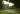The average SAT score of 1380 breaks down into:

• SAT math: 690

The average ACT score for Kenyon College is 31 and their acceptance rate is 35.8%.

## Ohio State University SAT scores

The average SAT score for Ohio State University is 1355.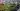The average SAT score of 1355 breaks down into:

• SAT math: 710

The average ACT score for Ohio State University is 30 and their acceptance rate is 53.7%.

## Miami University SAT scores

The average SAT score for Miami University is 1310.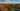The average SAT score of 1310 breaks down into:

• SAT math: 670

The average ACT score for Miami University is 29 and their acceptance rate is 83.8%.

## Denison University SAT scores

The average SAT score for Denison University is 1300.The average SAT score of 1300 breaks down into:

• SAT math: 660

The average ACT score for Denison University is 30 and their acceptance rate is 33.9%.

## The College of Wooster SAT scores

The average SAT score for The College of Wooster is 1268.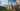The average SAT score of 1268 breaks down into:

• SAT math: 640

The average ACT score for The College of Wooster is 27 and their acceptance rate is 56.4%.

## University of Cincinnati SAT scores

The average SAT score for University of Cincinnati is 1265.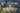The average SAT score of 1265 breaks down into:

• SAT math: 640

The average ACT score for University of Cincinnati is 27 and their acceptance rate is 73.1%.

## Cedarville University SAT scores

The average SAT score for Cedarville University is 1242.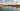The average SAT score of 1242 breaks down into:

• SAT math: 610

The average ACT score for Cedarville University is 26 and their acceptance rate is 79.4%.

## Otterbein University SAT scores

The average SAT score for Otterbein University is 1210.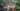The average SAT score of 1210 breaks down into:

• SAT math: 600

The average ACT score for Otterbein University is 23 and their acceptance rate is 82.5%.

## Ohio Northern University SAT scores

The average SAT score for Ohio Northern University is 1200.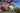The average SAT score of 1200 breaks down into:

• SAT math: 610